Confidence Intervals

# 42 Calculating the Sample Size n: Continuous and Binary Random Variables

Continuous Random VariablesUsually we have no control over the sample size of a data set. However, if we are able to set the sample size, as in cases where we are taking a survey, it is very helpful to know just how large it should be to provide the most information. Sampling can be very costly in both time and product. Simple telephone surveys will cost approximately ?30.00 each, for example, and some sampling requires the destruction of the product.

If we go back to our standardizing formula for the sampling distribution for means, we can see that it is possible to solve it for n. If we do this we have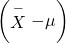in the denominator.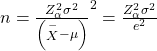Because we have not taken a sample yet we do not know any of the variables in the formula except that we can set Zα to the level of confidence we desire just as we did when determining confidence intervals. If we set a predetermined acceptable error, or tolerance, for the difference between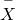and μ, called e in the formula, we are much further in solving for the sample size n. We still do not know the population standard deviation, σ. In practice, a pre-survey is usually done which allows for fine tuning the questionnaire and will give a sample standard deviation that can be used. In other cases, previous information from other surveys may be used for σ in the formula. While crude, this method of determining the sample size may help in reducing cost significantly. It will be the actual data gathered that determines the inferences about the population, so caution in the sample size is appropriate calling for high levels of confidence and small sampling errors.

Binary Random VariablesWhat was done in cases when looking for the mean of a distribution can also be done when sampling to determine the population parameter p for proportions. Manipulation of the standardizing formula for proportions gives: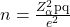where e = (p′-p), and is the acceptable sampling error, or tolerance, for this application. This will be measured in percentage points.

In this case the very object of our search is in the formula, p, and of course q because q =1-p. This result occurs because the binomial distribution is a one parameter distribution. If we know p then we know the mean and the standard deviation. Therefore, p shows up in the standard deviation of the sampling distribution which is where we got this formula. If, in an abundance of caution, we substitute 0.5 for p we will draw the largest required sample size that will provide the level of confidence specified by Zα and the tolerance we have selected. This is true because of all combinations of two fractions that add to one, the largest multiple is when each is 0.5. Without any other information concerning the population parameter p, this is the common practice. This may result in oversampling, but certainly not under sampling, thus, this is a cautious approach.

There is an interesting trade-off between the level of confidence and the sample size that shows up here when considering the cost of sampling. (Figure) shows the appropriate sample size at different levels of confidence and different level of the acceptable error, or tolerance.

Required sample size (90%) Required sample size (95%) Tolerance level
1691 2401 2%
752 1067 3%
271 384 5%
68 96 10%

This table is designed to show the maximum sample size required at different levels of confidence given an assumed p= 0.5 and q=0.5 as discussed above.

The acceptable error, called tolerance in the table, is measured in plus or minus values from the actual proportion. For example, an acceptable error of 5% means that if the sample proportion was found to be 26 percent, the conclusion would be that the actual population proportion is between 21 and 31 percent with a 90 percent level of confidence if a sample of 271 had been taken. Likewise, if the acceptable error was set at 2%, then the population proportion would be between 24 and 28 percent with a 90 percent level of confidence, but would require that the sample size be increased from 271 to 1,691. If we wished a higher level of confidence, we would require a larger sample size. Moving from a 90 percent level of confidence to a 95 percent level at a plus or minus 5% tolerance requires changing the sample size from 271 to 384. A very common sample size often seen reported in political surveys is 384. With the survey results it is frequently stated that the results are good to a plus or minus 5% level of “accuracy”.

Suppose a mobile phone company wants to determine the current percentage of customers aged 50+ who use text messaging on their cell phones. How many customers aged 50+ should the company survey in order to be 90% confident that the estimated (sample) proportion is within three percentage points of the true population proportion of customers aged 50+ who use text messaging on their cell phones.

From the problem, we know that the acceptable error, e, is 0.03 (3%=0.03) and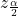z0.05 = 1.645 because the confidence level is 90%. The acceptable error, e, is the difference between the actual population proportion p, and the sample proportion we expect to get from the sample.

However, in order to find n, we need to know the estimated (sample) proportion p′. Remember that q′ = 1 – p′. But, we do not know p′ yet. Since we multiply p′ and q′ together, we make them both equal to 0.5 because pq′ = (0.5)(0.5) = 0.25 results in the largest possible product. (Try other products: (0.6)(0.4) = 0.24; (0.3)(0.7) = 0.21; (0.2)(0.8) = 0.16 and so on). The largest possible product gives us the largest n. This gives us a large enough sample so that we can be 90% confident that we are within three percentage points of the true population proportion. To calculate the sample size n, use the formula and make the substitutions.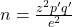gives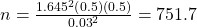Round the answer to the next higher value. The sample size should be 752 cell phone customers aged 50+ in order to be 90% confident that the estimated (sample) proportion is within three percentage points of the true population proportion of all customers aged 50+ who use text messaging on their cell phones.

Try It

Suppose an internet marketing company wants to determine the current percentage of customers who click on ads on their smartphones. How many customers should the company survey in order to be 90% confident that the estimated proportion is within five percentage points of the true population proportion of customers who click on ads on their smartphones?

271 customers should be surveyed.Check the Real Estate section in your local

### Chapter Review

Sometimes researchers know in advance that they want to estimate a population mean within a specific margin of error for a given level of confidence. In that case, solve the relevant confidence interval formula for n to discover the size of the sample that is needed to achieve this goal: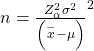If the random variable is binary then the formula for the appropriate sample size to maintain a particular level of confidence with a specific tolerance level is given by### Formula Review

n =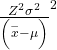= the formula used to determine the sample size (n) needed to achieve a desired margin of error at a given level of confidence for a continuous random variable= the formula used to determine the sample size if the random variable is binary

Use the following information to answer the next five exercises: The standard deviation of the weights of elephants is known to be approximately 15 pounds. We wish to construct a 95% confidence interval for the mean weight of newborn elephant calves. Fifty newborn elephants are weighed. The sample mean is 244 pounds. The sample standard deviation is 11 pounds.

Identify the following:

1.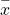= _____
2. σ = _____
3. n = _____
1. 244
2. 15
3. 50

In words, define the random variables X and.

Which distribution should you use for this problem?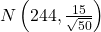Construct a 95% confidence interval for the population mean weight of newborn elephants. State the confidence interval, sketch the graph, and calculate the error bound.

What will happen to the confidence interval obtained, if 500 newborn elephants are weighed instead of 50? Why?

As the sample size increases, there will be less variability in the mean, so the interval size decreases.

Use the following information to answer the next seven exercises: The U.S. Census Bureau conducts a study to determine the time needed to complete the short form. The Bureau surveys 200 people. The sample mean is 8.2 minutes. There is a known standard deviation of 2.2 minutes. The population distribution is assumed to be normal.

Identify the following:

1.= _____
2. σ = _____
3. n = _____

In words, define the random variables X and.

X is the time in minutes it takes to complete the U.S. Census short form.is the mean time it took a sample of 200 people to complete the U.S. Census short form.

Which distribution should you use for this problem?

Construct a 90% confidence interval for the population mean time to complete the forms. State the confidence interval, sketch the graph, and calculate the error bound.

CI: (7.9441, 8.4559)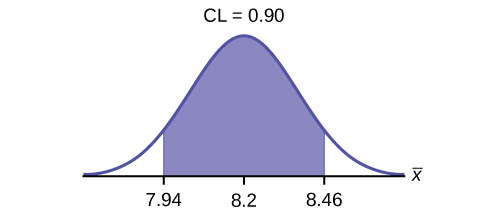If the Census wants to increase its level of confidence and keep the error bound the same by taking another survey, what changes should it make?

If the Census did another survey, kept the error bound the same, and surveyed only 50 people instead of 200, what would happen to the level of confidence? Why?

The level of confidence would decrease because decreasing n makes the confidence interval wider, so at the same error bound, the confidence level decreases.

Suppose the Census needed to be 98% confident of the population mean length of time. Would the Census have to survey more people? Why or why not?

Use the following information to answer the next ten exercises: A sample of 20 heads of lettuce was selected. Assume that the population distribution of head weight is normal. The weight of each head of lettuce was then recorded. The mean weight was 2.2 pounds with a standard deviation of 0.1 pounds. The population standard deviation is known to be 0.2 pounds.

Identify the following:

1.= ______
2. σ = ______
3. n = ______
1.= 2.2
2. σ = 0.2
3. n = 20

In words, define the random variable X.

In words, define the random variable.is the mean weight of a sample of 20 heads of lettuce.

Which distribution should you use for this problem?

Construct a 90% confidence interval for the population mean weight of the heads of lettuce. State the confidence interval, sketch the graph, and calculate the error bound.

EBM = 0.07
CI: (2.1264, 2.2736)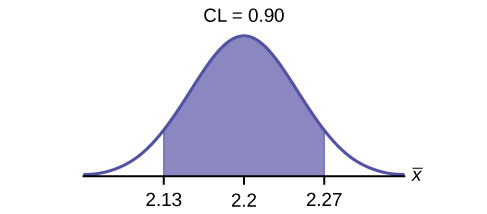Construct a 95% confidence interval for the population mean weight of the heads of lettuce. State the confidence interval, sketch the graph, and calculate the error bound.

In complete sentences, explain why the confidence interval in (Figure) is larger than in (Figure).

The interval is greater because the level of confidence increased. If the only change made in the analysis is a change in confidence level, then all we are doing is changing how much area is being calculated for the normal distribution. Therefore, a larger confidence level results in larger areas and larger intervals.

In complete sentences, give an interpretation of what the interval in (Figure) means.

What would happen if 40 heads of lettuce were sampled instead of 20, and the error bound remained the same?

The confidence level would increase.

What would happen if 40 heads of lettuce were sampled instead of 20, and the confidence level remained the same?

Use the following information to answer the next 14 exercises: The mean age for all Foothill College students for a recent Fall term was 33.2. The population standard deviation has been pretty consistent at 15. Suppose that twenty-five Winter students were randomly selected. The mean age for the sample was 30.4. We are interested in the true mean age for Winter Foothill College students. Let X = the age of a Winter Foothill College student.= _____

30.4

n = _____

________ = 15

σ

In words, define the random variable.

What isestimating?

μ

Is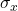known?

As a result of your answer to (Figure), state the exact distribution to use when calculating the confidence interval.

normal

Construct a 95% Confidence Interval for the true mean age of Winter Foothill College students by working out then answering the next seven exercises.

How much area is in both tails (combined)? α =________

How much area is in each tail?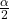=________

0.025

Identify the following specifications:

1. lower limit
2. upper limit
3. error bound

The 95% confidence interval is:__________________.

(24.52,36.28)

Fill in the blanks on the graph with the areas, upper and lower limits of the confidence interval, and the sample mean.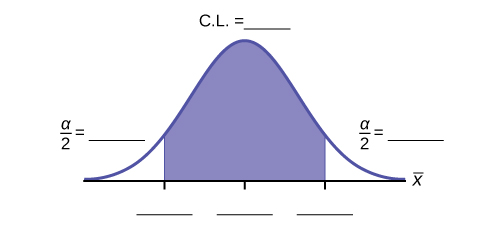In one complete sentence, explain what the interval means.

We are 95% confident that the true mean age for Winger Foothill College students is between 24.52 and 36.28.

Using the same mean, standard deviation, and level of confidence, suppose that n were 69 instead of 25. Would the error bound become larger or smaller? How do you know?

Using the same mean, standard deviation, and sample size, how would the error bound change if the confidence level were reduced to 90%? Why?

The error bound for the mean would decrease because as the CL decreases, you need less area under the normal curve (which translates into a smaller interval) to capture the true population mean.

Find the value of the sample size needed to if the confidence interval is 90% that the sample proportion and the population proportion are within 4% of each other. The sample proportion is 0.60. Note: Round all fractions up for n.

Find the value of the sample size needed to if the confidence interval is 95% that the sample proportion and the population proportion are within 2% of each other. The sample proportion is 0.650. Note: Round all fractions up for n.

2,185

Find the value of the sample size needed to if the confidence interval is 96% that the sample proportion and the population proportion are within 5% of each other. The sample proportion is 0.70. Note: Round all fractions up for n.

Find the value of the sample size needed to if the confidence interval is 90% that the sample proportion and the population proportion are within 1% of each other. The sample proportion is 0.50. Note: Round all fractions up for n.

6,765

Find the value of the sample size needed to if the confidence interval is 94% that the sample proportion and the population proportion are within 2% of each other. The sample proportion is 0.65. Note: Round all fractions up for n.

Find the value of the sample size needed to if the confidence interval is 95% that the sample proportion and the population proportion are within 4% of each other. The sample proportion is 0.45. Note: Round all fractions up for n.

595

Find the value of the sample size needed to if the confidence interval is 90% that the sample proportion and the population proportion are within 2% of each other. The sample proportion is 0.3. Note: Round all fractions up for n.

### Homework

Among various ethnic groups, the standard deviation of heights is known to be approximately three inches. We wish to construct a 95% confidence interval for the mean height of male Swedes. Forty-eight male Swedes are surveyed. The sample mean is 71 inches. The sample standard deviation is 2.8 inches.

1.=________
2. σ =________
3. n =________
1. In words, define the random variables X and.
2. Which distribution should you use for this problem? Explain your choice.
3. Construct a 95% confidence interval for the population mean height of male Swedes.
1. State the confidence interval.
2. Sketch the graph.
4. What will happen to the level of confidence obtained if 1,000 male Swedes are surveyed instead of 48? Why?
1. 71
2. 2.8
3. 48
1. X is the height of a male Swede, and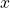is the mean height from a sample of 48 male Swedes.
2. Normal. We know the standard deviation for the population, and the sample size is greater than 30.
1. CI: (70.151, 71.85)
2.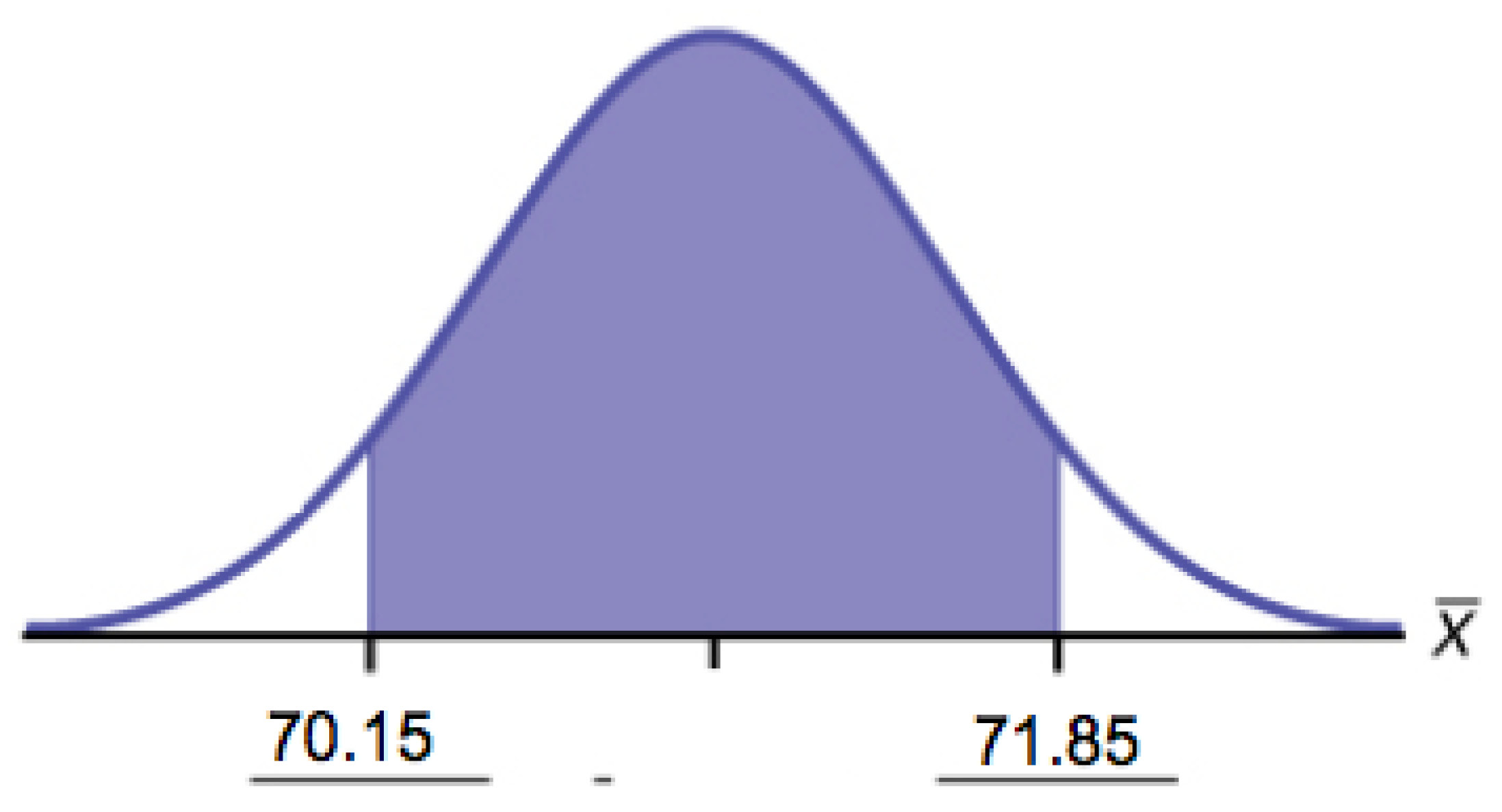3. The confidence interval will decrease in size, because the sample size increased. Recall, when all factors remain unchanged, an increase in sample size decreases variability. Thus, we do not need as large an interval to capture the true population mean.

Announcements for 84 upcoming engineering conferences were randomly picked from a stack of IEEE Spectrum magazines. The mean length of the conferences was 3.94 days, with a standard deviation of 1.28 days. Assume the underlying population is normal.

1. In words, define the random variables X and.
2. Which distribution should you use for this problem? Explain your choice.
3. Construct a 95% confidence interval for the population mean length of engineering conferences.
1. State the confidence interval.
2. Sketch the graph.

Suppose that an accounting firm does a study to determine the time needed to complete one person’s tax forms. It randomly surveys 100 people. The sample mean is 23.6 hours. There is a known standard deviation of 7.0 hours. The population distribution is assumed to be normal.

1.=________
2. σ =________
3. n =________
1. In words, define the random variables X and.
2. Which distribution should you use for this problem? Explain your choice.
3. Construct a 90% confidence interval for the population mean time to complete the tax forms.
1. State the confidence interval.
2. Sketch the graph.
4. If the firm wished to increase its level of confidence and keep the error bound the same by taking another survey, what changes should it make?
5. If the firm did another survey, kept the error bound the same, and only surveyed 49 people, what would happen to the level of confidence? Why?
6. Suppose that the firm decided that it needed to be at least 96% confident of the population mean length of time to within one hour. How would the number of people the firm surveys change? Why?
1.= 23.6
2.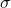= 7
3. n = 100
1. X is the time needed to complete an individual tax form.is the mean time to complete tax forms from a sample of 100 customers.
2.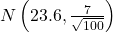because we know sigma.
1. (22.228, 24.972)
2.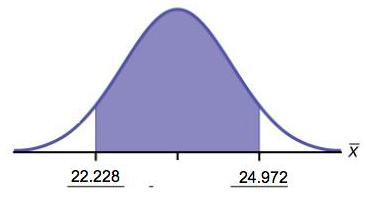3. It will need to change the sample size. The firm needs to determine what the confidence level should be, then apply the error bound formula to determine the necessary sample size.
4. The confidence level would increase as a result of a larger interval. Smaller sample sizes result in more variability. To capture the true population mean, we need to have a larger interval.
5. According to the error bound formula, the firm needs to survey 206 people. Since we increase the confidence level, we need to increase either our error bound or the sample size.

A sample of 16 small bags of the same brand of candies was selected. Assume that the population distribution of bag weights is normal. The weight of each bag was then recorded. The mean weight was two ounces with a standard deviation of 0.12 ounces. The population standard deviation is known to be 0.1 ounce.

1.=________
2. σ =________
3. sx =________
1. In words, define the random variable X.
2. In words, define the random variable.
3. Which distribution should you use for this problem? Explain your choice.
4. Construct a 90% confidence interval for the population mean weight of the candies.
1. State the confidence interval.
2. Sketch the graph.
5. Construct a 98% confidence interval for the population mean weight of the candies.
1. State the confidence interval.
2. Sketch the graph.
3. Calculate the error bound.
6. In complete sentences, explain why the confidence interval in part f is larger than the confidence interval in part e.
7. In complete sentences, give an interpretation of what the interval in part f means.

A camp director is interested in the mean number of letters each child sends during his or her camp session. The population standard deviation is known to be 2.5. A survey of 20 campers is taken. The mean from the sample is 7.9 with a sample standard deviation of 2.8.

1.=________
2. σ =________
3. n =________
1. Define the random variables X andin words.
2. Which distribution should you use for this problem? Explain your choice.
3. Construct a 90% confidence interval for the population mean number of letters campers send home.
1. State the confidence interval.
2. Sketch the graph.
4. What will happen to the error bound and confidence interval if 500 campers are surveyed? Why?
1. 7.9
2. 2.5
3. 20
1. X is the number of letters a single camper will send home.is the mean number of letters sent home from a sample of 20 campers.
2. N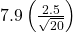1. CI: (6.98, 8.82)
2.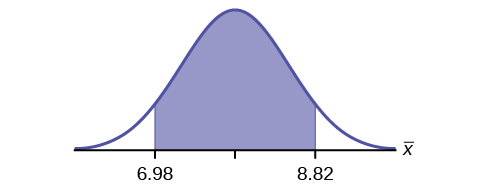3. The error bound and confidence interval will decrease.

What is meant by the term “90% confident” when constructing a confidence interval for a mean?

1. If we took repeated samples, approximately 90% of the samples would produce the same confidence interval.
2. If we took repeated samples, approximately 90% of the confidence intervals calculated from those samples would contain the sample mean.
3. If we took repeated samples, approximately 90% of the confidence intervals calculated from those samples would contain the true value of the population mean.
4. If we took repeated samples, the sample mean would equal the population mean in approximately 90% of the samples.

The Federal Election Commission collects information about campaign contributions and disbursements for candidates and political committees each election cycle. During the 2012 campaign season, there were 1,619 candidates for the House of Representatives across the United States who received contributions from individuals. (Figure) shows the total receipts from individuals for a random selection of 40 House candidates rounded to the nearest ?100. The standard deviation for this data to the nearest hundred is σ = ?909,200.

 ?3,600 ?1,243,900 ?10,900 ?385,200 ?581,500 ?7,400 ?2,900 ?400 ?3,714,500 ?632,500 ?391,000 ?467,400 ?56,800 ?5,800 ?405,200 ?733,200 ?8,000 ?468,700 ?75,200 ?41,000 ?13,300 ?9,500 ?953,800 ?1,113,500 ?1,109,300 ?353,900 ?986,100 ?88,600 ?378,200 ?13,200 ?3,800 ?745,100 ?5,800 ?3,072,100 ?1,626,700 ?512,900 ?2,309,200 ?6,600 ?202,400 ?15,800
1. Find the point estimate for the population mean.
2. Using 95% confidence, calculate the error bound.
3. Create a 95% confidence interval for the mean total individual contributions.
4. Interpret the confidence interval in the context of the problem.
1.= ?568,873
2. CL = 0.95 α = 1 – 0.95 = 0.05= 1.96
EBM =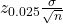= 1.96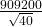= ?281,764
3.EBM = 568,873 − 281,764 = 287,109+ EBM = 568,873 + 281,764 = 850,637
4. We estimate with 95% confidence that the mean amount of contributions received from all individuals by House candidates is between ?287,109 and ?850,637.

The American Community Survey (ACS), part of the United States Census Bureau, conducts a yearly census similar to the one taken every ten years, but with a smaller percentage of participants. The most recent survey estimates with 90% confidence that the mean household income in the U.S. falls between ?69,720 and ?69,922. Find the point estimate for mean U.S. household income and the error bound for mean U.S. household income.

The average height of young adult males has a normal distribution with standard deviation of 2.5 inches. You want to estimate the mean height of students at your college or university to within one inch with 93% confidence. How many male students must you measure?

If the confidence interval is change to a higher probability, would this cause a lower, or a higher, minimum sample size?

Higher

If the tolerance is reduced by half, how would this affect the minimum sample size?

It would increase to four times the prior value.

If the value of p is reduced, would this necessarily reduce the sample size needed?

No, It could have no affect if it were to change to 1 – p, for example. If it gets closer to 0.5 the minimum sample size would increase.

Is it acceptable to use a higher sample size than the one calculated by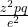?

Yes

A company has been running an assembly line with 97.42%% of the products made being acceptable. Then, a critical piece broke down. After the repairs the decision was made to see if the number of defective products made was still close enough to the long standing production quality. Samples of 500 pieces were selected at random, and the defective rate was found to be 0.025%.

1. Is this sample size adequate to claim the company is checking within the 90% confidence interval?
2. The 95% confidence interval?
1. No
2. No